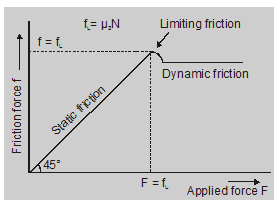Download the eSaral app and start learning from Kota's top IITians and doctors.

# What is Static Friction - Friction, Physics, Class 11 - eSaral

Hey, do you want to know what is Static Friction? If yes. Then keep reading.

## Static friction

The frictional force between two surfaces before the relative motion actually starts is called static frictional force or static friction. If we slowly increase the force with which we are pulling the box, the graph shows that the friction force increases with our force up to a certain critical value, $\mathrm{f}_{\mathrm{L}}$, the box suddenly begins to move, and as soon as it starts moving, a smaller force is required to maintain its motion as in motion friction is reduced. The friction value from 0 to $\mathrm{f}_{\mathrm{L}}$ is known as static friction, which balances the external force on the body and prevents it from sliding. The value $\mathrm{f}_{\mathrm{L}}$ is the maximum limit up to which the static friction acts is known as limiting friction, after which the body starts sliding and friction reduces to kinetic friction.## Laws of Static Friction

1. The direction of the force of friction is always in a direction opposite to which the body moves or tends to move.
2. The force of friction is a self-adjusting force and increases with the applied force, so as to be equal and opposite to it until the motion is just about to start.
3. When one body just tends to move, the force of friction between the surfaces in contact is maximum. The force of friction is called limiting friction.
4. The limiting friction depends on the nature of surfaces in contact but is independent of the area of the surface in contact.
5. The limiting friction between the surface of two bodies is directly proportional to the normal reaction N i.e. $F_{s} \propto N$.
6. The frictional force is independent of the relative velocities of the two surfaces.

## Coefficient of Static Friction $\left(\mu_{s}\right)$

The limiting friction $\mathrm{F}_{\mathrm{S}}$ is proportional to the normal reaction N thus

$\mathrm{F}_{\mathrm{s}}=\mu_{\mathrm{S}} \mathrm{N}$

Or

$\mu_{S}=\frac{F_{s}}{N}$

$=\frac{\text { limiting friction }}{\text { normal reaction }}$

so, that's all from this blog. I hope you get the idea about What is Static Friction/ If you liked this Explanation then please share it with your friends.

If you have any confusion related to this blog then feel free to ask in the comments section down below.